MORE IN Basic Electrical & Electronics Engg
MU First Year Engineering (Semester 1)
Basic Electrical & Electronics Engg
May 2014
Total marks: --
Total time: --
INSTRUCTIONS
(1) Assume appropriate data and state your reasons
(2) Marks are given to the right of every question
(3) Draw neat diagrams wherever necessary

1 (a) Using source conversion, reduce the circuit shown in figure into single current source in parallel with single resistance.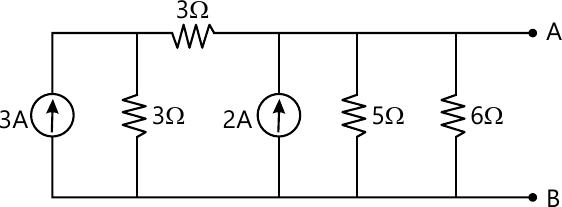3 M
1 (b) Derive the condition for maximum power transfer through the network.
3 M
1 (c) An alternating current takes 3.375 ms to reach 15A for the first time after becoming instantaneously zero. The Frequency of the current is 40 Hz. Find the Maximum value of the alternating current.
3 M
1 (d) Derive the equation for resonance frequency [fr] in parallel resonance circuit.
3 M
1 (e) Three identical coils each [4.2-j5.6] ohm are connected in star Across 415 V, 3 Phase, 50Hz supply. Deterine (i) Vph (ii) Iph (iii) Power factor.
2 M
1 (f) What are the losses in the transformer? Explain why the rating of transformer in KVA not in KW.
4 M
1 (g) Draw complete V.I characteristics of a Diode.
2 M

2 (a) Determine the potential different VAB for the given network.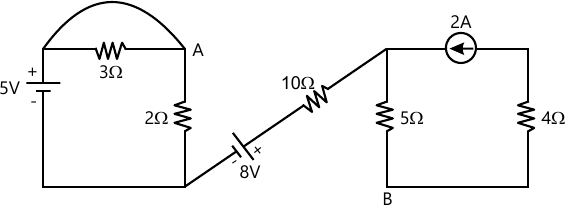6 M
2 (b) When a resistor and an inductor in series are connected to a 240V supply, a current of 3A flows lagging 37o behind the supply voltage, while voltage across inductor is 171 volt. Find the resistance of resistor, resistance & reactance of the inductor.
8 M
2 (c) Draw phasor diagram of single phase transformer on resistive load [Unity power factor] and inductive load [lagging power factor].
6 M

3 (a) Three similar coils connected in star, take a total power of 18KW at a power factor of 0.866 lagging from a three phase 400 volts. 50 Hz system. Calculate the resistance and inductance of each coil.Also draw the phasor diagram showing the current and voltages.
8 M
3 (b) A 5 KVA 200/400 volts, 50Hz single phase transformer gave the following test results.
O.C. test [LV side] 200V 0.7A 60W
S.C. test [HV side] 22V 16V 120W
(i) Draw the equivalent circuit of the transformer referred to LV side insert all parameter values.
(ii) Efficiency at 0.9 power factor leading if operating at rated load.
6 M
3 (c) What is function of filter in rectifier circuit ? Draw of rectifire with inductor filter.
2 M
3 (d) Explain with circuit diagram working of CE configuration of BJT.
4 M

4 (a) Find a equivalent resistance between A and B.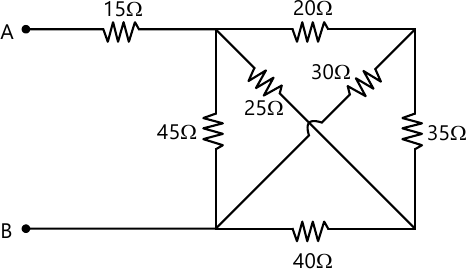7 M
4 (b) A circuit consists of three parallel branches. The branch current are given as $f_1=10Sin\ \omega{}t,\ i_2=20\ Sin\ \left(\omega{}t+60^\circ{}\right),\ and\ i_3=75Sin\ \left(\omega{}t-30\right).\ \$ Find the resultant current and express it in the form i=Im Sin (Ωt+θ). if the supply frequency is 50Hz, calculate the resultant current when (i) t=0, (ii) t=0.001 sec
5 M
4 (c) A 3 phase, 10 KVA lead has power factor of 0.342.The power is measured by two wattmeter method. Find the reading of each wattmeter when.
4 M
4 (d) Explain working of centre tap full wave rectifier with waveforms.
4 M

5 (a) Find the current through 60Ω resistance by using Thevenin's theorem.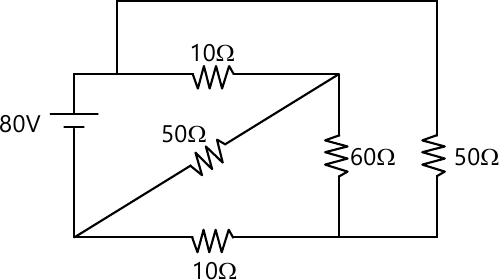8 M
5 (b) Find current I1 and I2 shown in figure.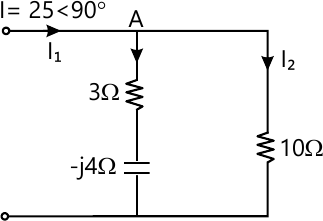4 M
5 (c) A 50KVA, 4400/220 volt transformer has R1 =3.45Ω, R2 =0.009Ω. The reactance are X1=5.2Ω and X2=0.015Ω. Calculate for the transformer,
(i) Full load currents on primary and secondary side,
(ii) Equivalent resistance, rectances, impedances, referred to primary side and secondary side, and
(iii) Total copper lose using individual resistances and equivalent resistances.
8 M

6 (a) Find the current through 6Ω resistor using superposition theorem.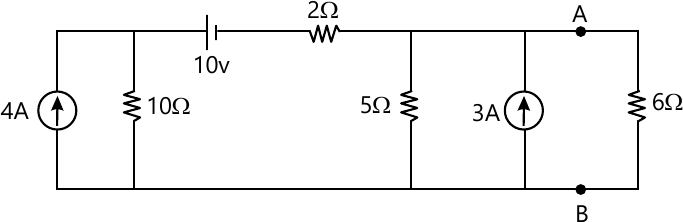7 M
6 (b) A coil of inductance 31.8mH with resistance of 12Ω is connected in parallel in with a capacitor across 250 volts, 50Hz supply. Determine the value of capacitance, if no reactive current is taken from the supply.
7 M
6 (c) Explain Measurement of three phase power using two wattmeter method.
6 M

More question papers from Basic Electrical & Electronics Engg